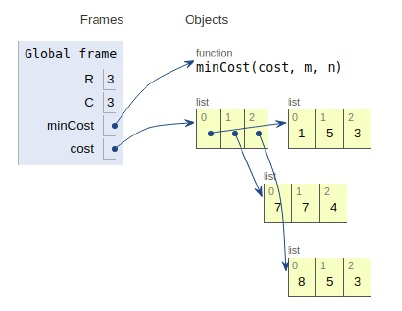# Python Program for Min Cost Path

Problem statement − We are given a cost matrix and a position (m, n), we need to find the cost of minimum cost path to reach (m, n) from (0, 0). Each cell represents a cost to traverse from one cell to another.

Now let’s observe the solution in the implementation below −

## Example

Live Demo

# dynamic approach
R = 3
C = 3
def minCost(cost, m, n):
# initialization
tc = [[0 for x in range(C)] for x in range(R)]
# base case
tc = cost
# total cost(tc) array
for i in range(1, m + 1):
tc[i] = tc[i-1] + cost[i]
# tc array
for j in range(1, n + 1):
tc[j] = tc[j-1] + cost[j]
# rest tc array
for i in range(1, m + 1):
for j in range(1, n + 1):
tc[i][j] = min(tc[i-1][j-1], tc[i-1][j], tc[i][j-1]) + cost[i][j]
return tc[m][n]
# main
cost = [[1, 5, 3],
[7, 7, 4],
[8, 5, 3]]
print("Total Cost:",minCost(cost, 2, 1))

## Output

Total Cost: 13All the variables are declared in the local scope and their references are seen in the figure above.

## Conclusion

In this article, we have learned about how we can make a Python Program for Min Cost Path